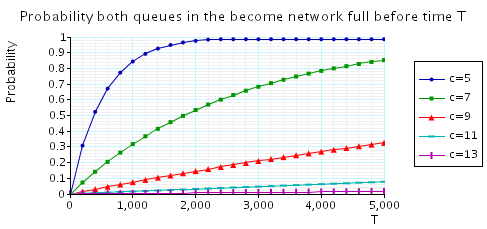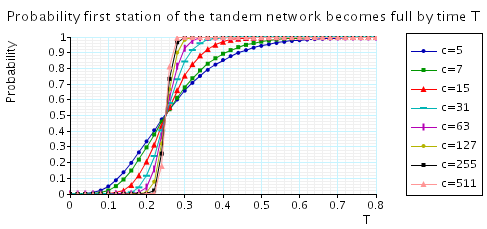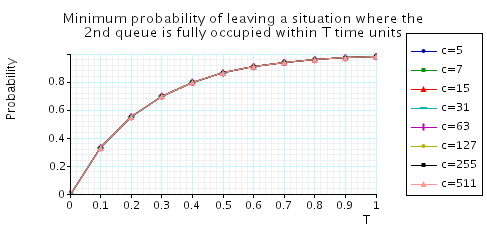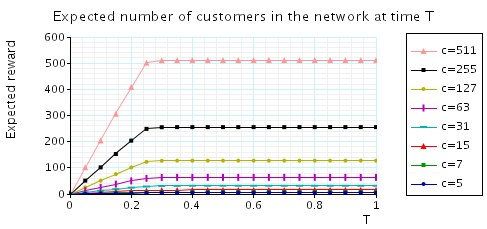# Tandem Queueing Network

(Hermanns, Meyer-Kayser & Siegle)

### Introduction

This case study is based on a simple tandem queueing network, taken from [HMKS99]. The model is represented as a CTMC which consists of a M/Cox2/1-queue sequentially composed with a M/M/1-queue. We use c to denote the capacity of the queues. The PRISM source code is given below.

```// tandem queueing network [HKMKS99]
// gxn/dxp 25/01/00

ctmc

const int c; // queue capacity

const double lambda = 4*c;
const double mu1a = 0.1*2;
const double mu1b = 0.9*2;
const double mu2 = 2;
const double kappa = 4;

module serverC

sc : [0..c];
ph : [1..2];

[] (sc<c) -> lambda: (sc'=sc+1);
[route] (sc>0) & (ph=1) -> mu1b: (sc'=sc-1);
[] (sc>0) & (ph=1) -> mu1a: (ph'=2);
[route] (sc>0) & (ph=2) -> mu2: (ph'=1) & (sc'=sc-1);

endmodule

module serverM

sm : [0..c];

[route]	(sm<c) -> 1: (sm'=sm+1);
[] (sm>0) -> kappa: (sm'=sm-1);

endmodule

// reward - number of customers in network
rewards "customers"
true : sc + sm;
endrewards
```

### Model Statistics

The table below shows statistics for each model we have built. The first section gives information about the CTMC representing the model: the number of states and the number of non-zeros (transitions). The second part refers to the MTBDD which represents this CTMC: the total number of nodes, the number of leaves (terminal nodes), and the amount of memory needed to store it. The last column gives the amount of memory a sparse matrix would need to represent the same CTMC.

Kb:
 c: Model: MTBDD: Sparse: States: NNZ: Nodes: Leaves: Kb: 5 66 189 100 6 2.0 2.73 7 120 363 93 6 1.8 5.19 15 496 1,619 123 6 2.4 22.8 31 2,016 6,819 153 6 3.0 95.6 63 8,128 27,971 183 6 3.6 391 127 32,640 113,283 213 6 4.2 1,582 255 130,816 455,939 243 6 4.7 6,365 511 523,776 1,829,379 273 6 5.3 25,530 1,023 2,096,128 7,328,771 303 6 5.9 102,260 2,047 8,386,560 29,337,603 333 6 6.5 409,320 4,095 33,550,336 117,395,459 363 6 7.1 1,637,840

### Model Construction Times

The table below gives the times taken to construct the models. This process involves first building a CTMC (represented as an MTBDD) from the system description (in our module based language), and then computing the reachable states using a BDD based fixpoint algorithm. The total time required and the number of fixpoint iterations are given below.

 c: Construction: Time (s): Iter.s: 5 0.034 17 7 0.035 23 15 0.040 47 31 0.058 95 63 0.128 191 127 0.374 383 255 1.409 767 511 5.565 1,535 1,023 22.70 3,071 2,047 99.64 6,143 4,095 763.6 12,287

### Model Checking

We now report on the model checking results obtained using PRISM when verifying the following CSL properties.

```const double T;

// long run expected customers in the network
R=?[S]

// probability network becomes full in T time units
P=? [ true U<=T sc=c & sm=c & ph=2 ]

// probability first queue becomes full in T time units
P=? [ true U<=T sc=c ]

// the minimimum probability of leaving a situation where the second queue is entirely populated within T time units
P=? [ sm=c U<=T sm<c {sm=c}{min} ]

// expected number of customers in the network
R=?[I=T]
```

All experiments were carried out on a 2.80GHz PC with 1 Gb memory running Linux and in all iterative methods we stop iterating when the relative error between subsequent iteration vectors is less than 1e-6, that is:• Computing Steady State Probabilities: The table below gives the model checking statistics when computing these probabilities for both the Jacobi and Gauss-Seidel methods when using PRISM's different engines.

 c: Jacobi: Gauss Seidel: Iters: Time per iter: (s) Iters: Time per iter: (s) MTBDD Sparse Hybrid Sparse Hybrid 5 189 0.001180 < 0.00001 < 0.00001 34 < 0.00001 < 0.00001 7 207 0.001791 < 0.00001 0.000010 54 < 0.00001 0.000037 15 298 0.006827 0.000013 0.000020 119 0.000034 0.000034 31 555 0.012806 0.000052 0.000074 218 0.000087 0.000188 63 1,056 0.051735 0.000227 0.000361 413 0.000363 0.000695 127 2,093 0.154552 0.003065 0.005955 816 0.004366 0.008109 255 4,212 0.224609 0.032259 0.041037 1,639 0.035350 0.045198 511 8,498 0.650746 0.166898 0.205874 3,303 0.176115 0.198061 1,023 16,326 1.092533 0.704615 0.789749 6,335 0.763633 0.817350 2,047 24,141 1.939870 2.877855 3.173416 9,394 3.085141 3.220208 4,095 37,931 - - 13.14700 15,189 - 14.38306

In the graph below we have plotted the results we checking the expected number of customers in the network in the long run.• The probability that, from the initial state, the tandem network becomes fully occupied within T time units. The table and graph below presents the statistics and results when verifying this property.

 c: T=2: Iters: Time per iter: (s) MTBDD Sparse Hybrid 5 225 0.001120 < 0.00001 < 0.00001 7 241 0.001780 0.000000 0.000008 15 306 0.008987 0.000016 0.000033 31 437 0.022659 0.000080 0.000135 63 723 0.042553 0.000372 0.000564 127 1,325 0.094658 0.002080 0.002654 255 2,483 0.256095 0.014267 0.027096 511 4,733 0.786130 0.045681 0.081702 1,023 9,137 2.564898 0.119471 0.254866 2,047 17,814 9.097987 0.433326 0.899096 4,095 34,980 - - 3.485094• The probability that, from the initial state, the first station of the tandem network becomes fully occupied within T time units. The table and graph below presents the statistics and results when verifying this property.

 c: T=0.5: Iters: Time per iter: (s) MTBDD Sparse Hybrid 5 30 0.000900 < 0.00001 < 0.00001 7 30 0.001433 < 0.00001 < 0.00001 15 35 0.004743 0.000057 0.000057 31 50 0.008660 0.000260 0.000280 63 82 0.011988 0.001354 0.001476 127 148 0.017135 0.006176 0.006743 255 281 0.021281 0.027103 0.029299 511 545 0.033506 0.111947 0.123903 1,023 1,073 0.044320 0.410707 0.488582 2,047 2,125 0.112301 1.647587 1.972453 4,095 4,225 0.149460 - 8.087538• The minimum probability of leaving a situation where the second queue is fully occupied within T time units. The table and graph below presents the statistics and results when verifying this property.

 c: T=0.5: Iters: Time per iter: (s) MTBDD Sparse Hybrid 5 69 0.000058 < 0.00001 < 0.00001 7 92 0.000098 < 0.00001 < 0.00001 15 176 0.000125 0.000011 < 0.00001 31 237 0.000139 0.000025 0.000030 63 302 0.000172 0.000086 0.000086 127 433 0.000219 0.000621 0.000672 255 718 0.000252 0.002249 0.002592 511 1,320 0.000309 0.010255 0.009667 1,023 2,479 0.000336 0.025934 0.041160 2,047 4,728 0.000355 0.098713 0.160120 4,095 9,133 0.000384 0.371677 0.626650• The expected number of customers in the network at time T. The table and graph below presents the statistics and results when verifying this property.

 c: T=1: Iters: Time per iter: (s) MTBDD Sparse Hybrid 5 198 0.001131 < 0.00001 < 0.00001 7 206 0.001801 0.000000 0.000010 15 239 0.006971 0.000017 0.000033 31 304 0.033102 0.000066 0.000109 63 435 0.075993 0.000260 0.000444 127 720 0.727625 0.001549 0.002162 255 1,323 1.212686 0.006620 0.009642 511 2,481 20.34883 0.026343 0.040297 1,023 1,063 - 0.067043 0.149812 2,047 2,111 - 0.267248 0.605698